Finding Minors and cofactors

Chapter 4 Class 12 Determinants
Concept wise

Let’s look at what are minors & cofactor of a 2 × 2 & a 3 × 3 determinant

## For a 2 × 2 determinant

For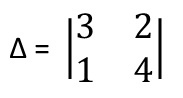We have elements,
𝑎 11 = 3
𝑎 12 = 2
𝑎 21 = 1
𝑎 22 = 4

Minor will be

𝑀 11 ,  𝑀 12 ,  𝑀 21 ,  𝑀 22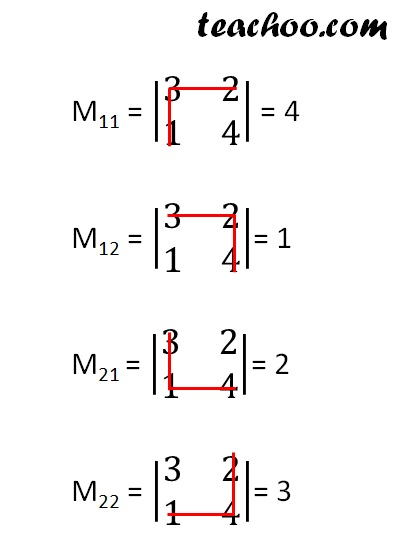And cofactors will be

𝐴 11 ,  𝐴 12 ,  𝐴 21 ,  𝐴 22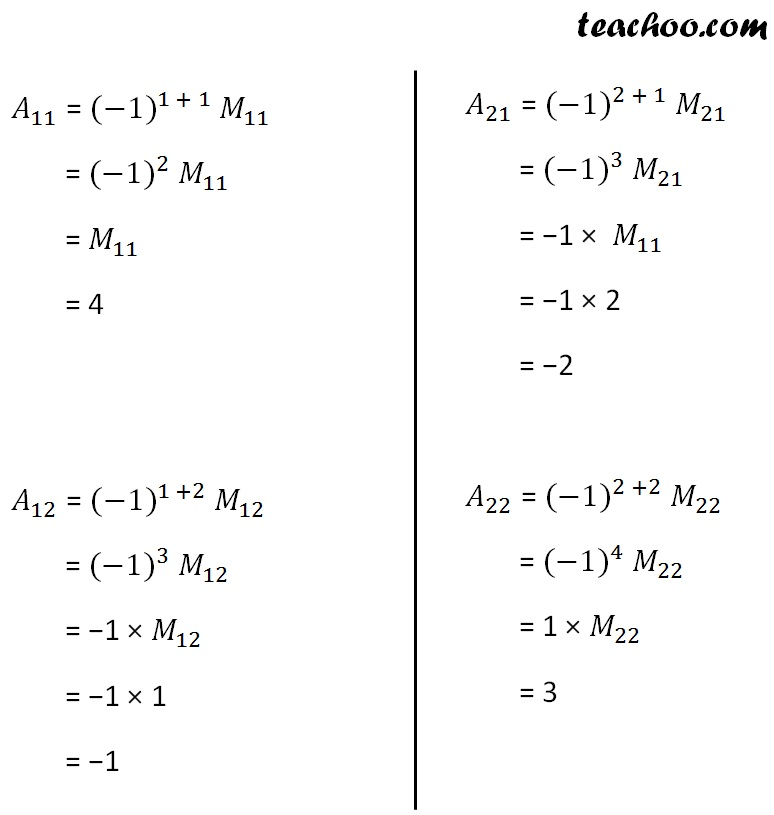## For a 3 × 3 matrix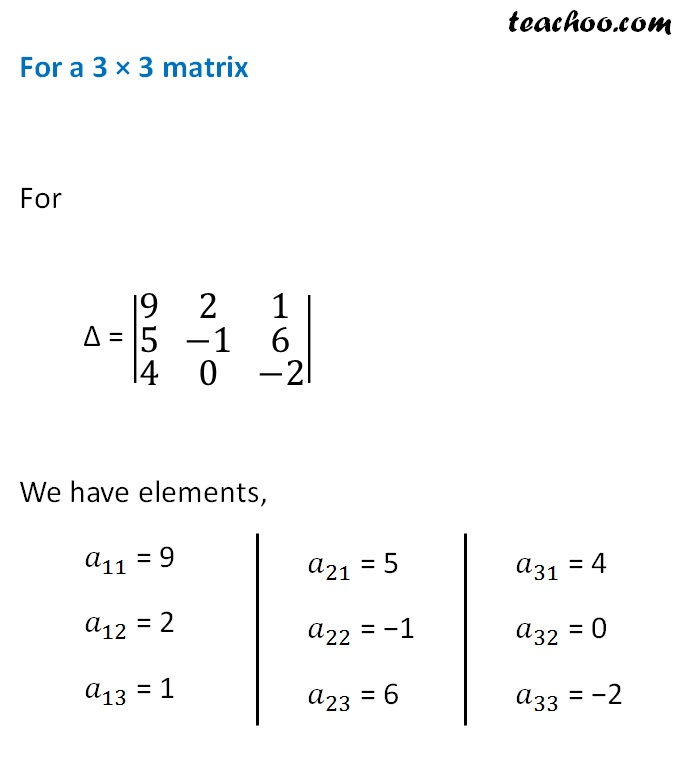Minor will be

M 11 , M 12 , M 13 , M 21 , M 22 , M 23 , M 31 , M 32 , M 33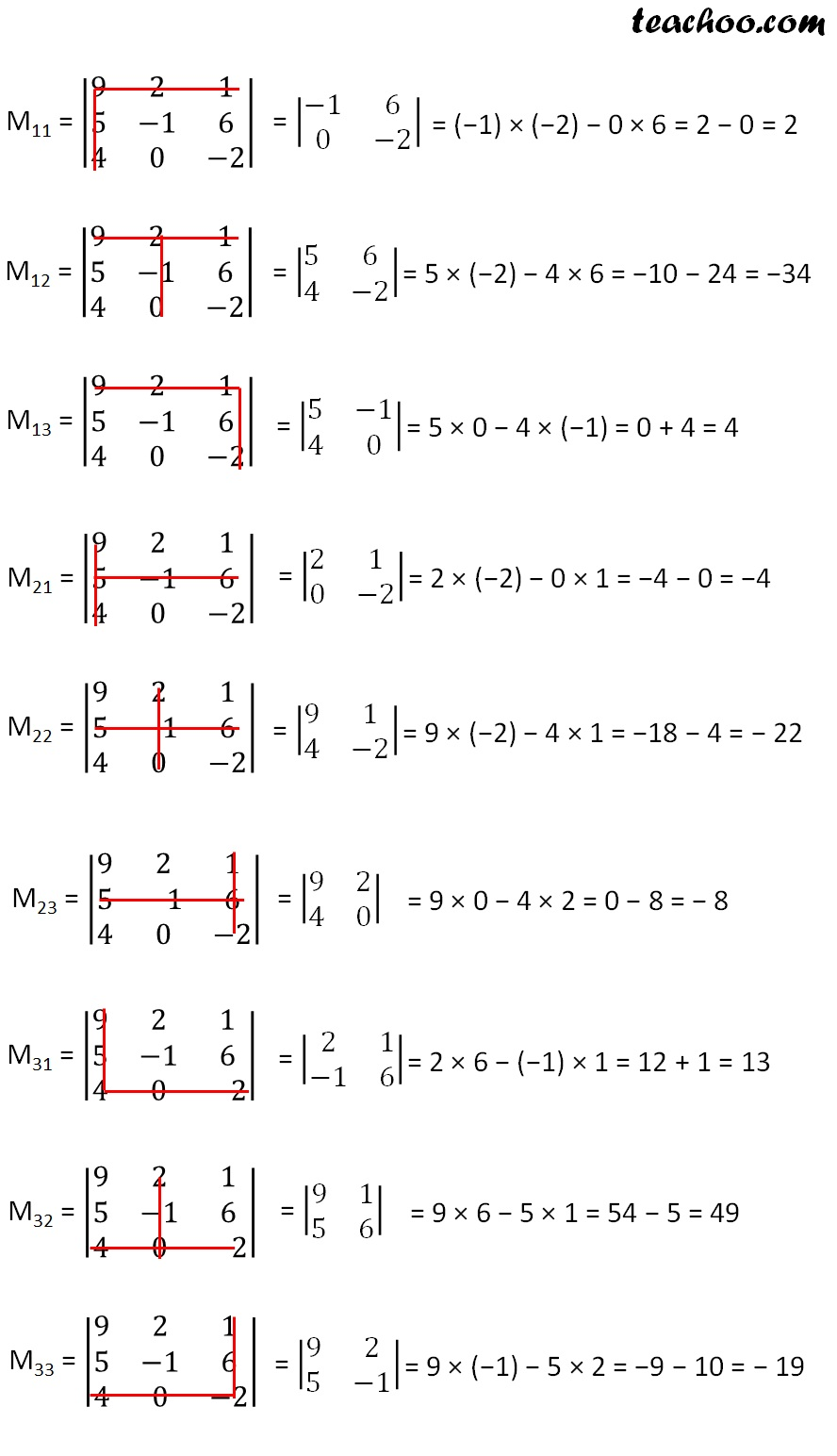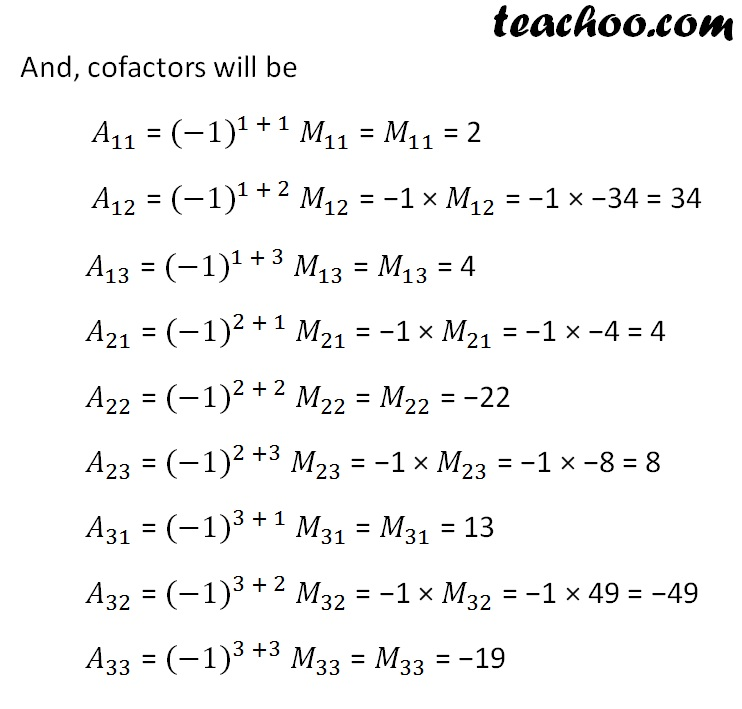Note : We can also calculate cofactors without calculating minors

If i + j is odd,

A ij = −1 × M ij

If i + j is even,

A ij = M ij

## But, why use cofactor?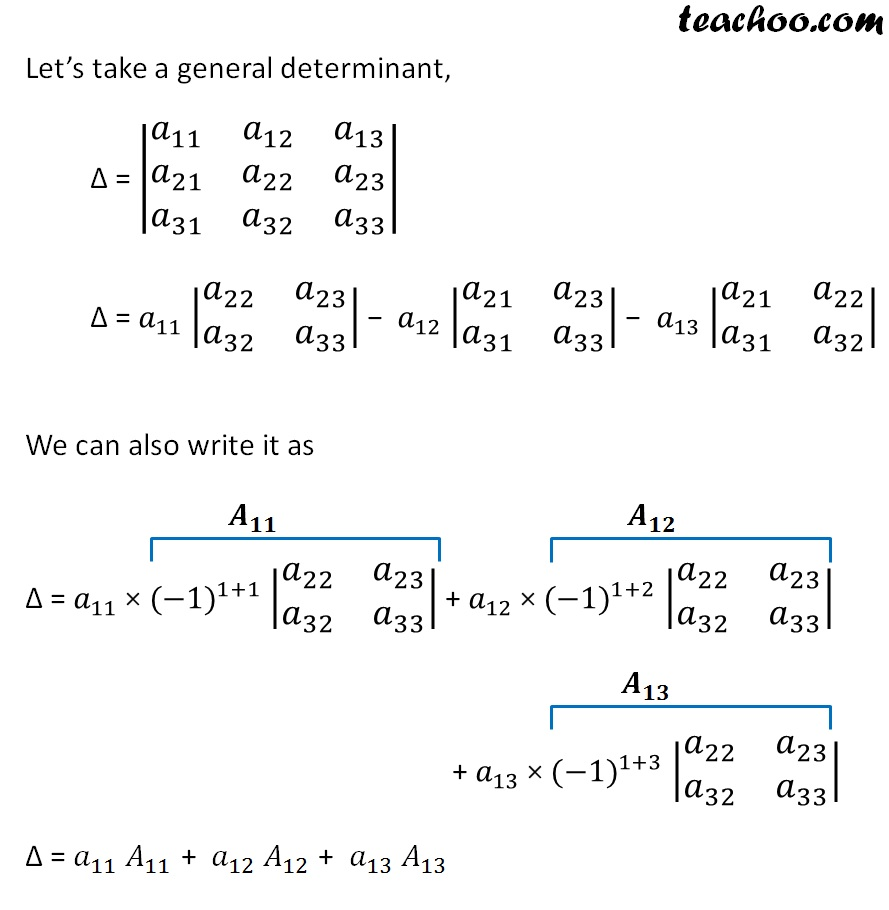Get live Maths 1-on-1 Classs - Class 6 to 12

### Transcript

For a 2 × 2 determinant For ∆ = |■8(3&[email protected]&4)| Minor will be 𝑀_11, 𝑀_12, 𝑀_21, 𝑀_22 M11 = |■8(3&[email protected]&4)| = 4 M12 = |■8(3&[email protected]&4)|= 1 M21 = |■8(3&[email protected]&4)|= 2 M22 = |■8(3&[email protected]&4)|= 3 And cofactors will be 𝐴_11, 𝐴_12, 𝐴_21, 𝐴_22 𝐴_11 = 〖(−1)〗^(1 + 1) 𝑀_11 = 〖(−1)〗^2 𝑀_11 = 𝑀_11 = 4 𝐴_12 = 〖(−1)〗^(1 +2) 𝑀_12 = 〖(−1)〗^3 𝑀_12 = −1 × 𝑀_12 = −1 × 1 = −1 𝐴_21 = 〖(−1)〗^(2 + 1) 𝑀_21 = 〖(−1)〗^3 𝑀_21 = −1 × 𝑀_11 = −1 × 2 = −2 𝐴_22 = 〖(−1)〗^(2 +2) 𝑀_22 = 〖(−1)〗^4 𝑀_22 = 1 × 𝑀_22 = 3 For a 3 × 3 matrix For ∆ = |■8(9&2&[email protected]&−1&[email protected]&0&−2)| We have elements, 𝑎_11 = 9 𝑎_12 = 2 𝑎_13 = 1 𝑎_21 = 5 𝑎_22 = −1 𝑎_23 = 6 𝑎_31 = 1 𝑎_32 = 6 𝑎_33 = −2 . Minor will be 𝑀_11, 𝑀_12, 𝑀_13, 𝑀_21, 𝑀_22, 𝑀_23, 𝑀_31, 𝑀_32, 𝑀_33 M11 = |■8(9&2&[email protected]&−1&[email protected]&0&−2)| = |■8(−1&[email protected]&−2)| = (−1) × (−2) − 0 × 6 = 2 − 0 = 2 M12 = |■8(9&2&[email protected]&−1&[email protected]&0&−2)| = |■8(5&[email protected]&−2)| = 5 × (−2) − 4 × 6 = −10 − 24 = −34 M13 = |■8(9&2&[email protected]&−1&[email protected]&0&−2)| = |■8(5&−[email protected]&0)| = 5 × 0 − 4 × (−1) = 0 + 4 = 4 M21 = |■8(9&2&[email protected]&−1&[email protected]&0&−2)| = |■8(2&[email protected]&−2)| = 2 × (−2) − 0 × 1 = −4 − 0 = −4 M22 = |■8(9&2&[email protected]&−1&[email protected]&0&−2)| = |■8(9&[email protected]&−2)| = 9 × (−2) − 4 × 1 = −18 − 4 = − 22 M23 = |■8(9&2&[email protected]&−1&[email protected]&0&−2)| = |■8(9&[email protected]&0)| = 9 × 0 − 4 × 2 = 0 − 8 = − 8 M31 = |■8(9&2&[email protected]&−1&[email protected]&0&−2)| = |■8(2&[email protected]−1&6)| = 2 × 6 − (−1) × 1 = 12 + 1 = 13 M32 = |■8(9&2&[email protected]&−1&[email protected]&0&−2)| = |■8(9&[email protected]&6)| = 9 × 6 − 5 × 1 = 54 − 5 = 49 M33 = |■8(9&2&[email protected]&−1&[email protected]&0&−2)| = |■8(9&[email protected]&−1)| = 9 × (−1) − 5 × 2 = −9 − 10 = − 19 And, cofactors will be 𝐴_11 = 〖(−1)〗^(1 + 1) 𝑀_11 = 𝑀_11 = 2 𝐴_12 = 〖(−1)〗^(1 + 2) 𝑀_12 = −1 × 𝑀_12 = −1 × −34 = 34 𝐴_13 = 〖(−1)〗^(1 + 3) 𝑀_13 = 𝑀_13 = 4 𝐴_21 = 〖(−1)〗^(2 + 1) 𝑀_21 = −1 × 𝑀_21 = −1 × −4 = 4 𝐴_22 = 〖(−1)〗^(2 + 2) 𝑀_22 = 𝑀_22 = −22 𝐴_23 = 〖(−1)〗^(2 +3) 𝑀_23 = −1 × 𝑀_23 = −1 × −8 = 8 𝐴_31 = 〖(−1)〗^(3 + 1) 𝑀_31 = 𝑀_31 = 13 𝐴_32 = 〖(−1)〗^(3 + 2) 𝑀_32 = −1 × 𝑀_32 = −1 × 49 = −49 𝐴_33 = 〖(−1)〗^(3 +3) 𝑀_33 = 𝑀_33 = −19 Note : We can also calculate cofactors without calculating minors If i + j is odd, Aij = −1 × Mij If i + j is even, Aij = Mij But, why use cofactor? Let’s take a general determinant, ∆ = |■8(𝑎_11&𝑎_12&𝑎[email protected]𝑎_21&𝑎_22&𝑎[email protected]𝑎_31&𝑎_32&𝑎_33 )| ∆ = 𝑎_11 |■8(𝑎_22&𝑎[email protected]𝑎_32&𝑎_33 )| − 𝑎_12 |■8(𝑎_21&𝑎[email protected]𝑎_31&𝑎_33 )| − 𝑎_13 |■8(𝑎_21&𝑎[email protected]𝑎_31&𝑎_32 )| We can also write it as ∆ = 𝑎_11 × 〖(−1)〗^(1+1) |■8(𝑎_22&𝑎[email protected]𝑎_32&𝑎_33 )| + 𝑎_12 × 〖(−1)〗^(1+2) |■8(𝑎_22&𝑎[email protected]𝑎_32&𝑎_33 )| + 𝑎_13 × 〖(−1)〗^(1+3) |■8(𝑎_22&𝑎[email protected]𝑎_32&𝑎_33 )| ∆ = 𝑎_11 𝐴_11 + 𝑎_12 𝐴_12 + 𝑎_13 𝐴_13